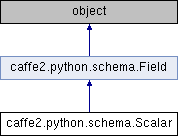Caffe2 - Python API A deep learning, cross platform ML framework
caffe2.python.schema.Scalar Class Reference
Inheritance diagram for caffe2.python.schema.Scalar:## Public Member Functions

def __init__ (self, dtype=None, blob=None, metadata=None)

def field_names (self)

def field_type (self)

def field_types (self)

def has_blobs (self)

def field_blobs (self)

def all_scalars (self)

def clone (self, keep_blobs=True)

def get (self)

def __call__ (self)

def set_value (self, blob, throw_on_type_mismatch=False, unsafe=False)

def set (self, dtype=None, blob=None, metadata=None, unsafe=False)

def set_type (self, dtype)

def id (self)Public Member Functions inherited from caffe2.python.schema.Field
def __init__ (self, children)

def clone_schema (self)

def field_names (self)

def field_types (self)

def field_blobs (self)

def all_scalars (self)

def has_blobs (self)

def clone (self, keep_blobs=True)

def slice (self)

def __eq__ (self, other)

def __repr__ (self)

dtype

## Detailed Description

```Represents a typed scalar or tensor of fixed shape.

A Scalar is a leaf in a schema tree, translating to exactly one tensor in
the dataset's underlying storage.

Usually, the tensor storing the actual values of this field is a 1D tensor,
representing a series of values in its domain. It is possible however to
have higher rank values stored as a Scalar, as long as all entries have
the same shape.

E.g.:

Scalar(np.float64)

Scalar field of type float64. Caffe2 will expect readers and
datasets to expose it as a 1D tensor of doubles (vector), where
the size of the vector is determined by this fields' domain.

Scalar((np.int32, 5))

Tensor field of type int32. Caffe2 will expect readers and
datasets to implement it as a 2D tensor (matrix) of shape (L, 5),
where L is determined by this fields' domain.

Scalar((str, (10, 20)))

Tensor field of type str. Caffe2 will expect readers and
datasets to implement it as a 3D tensor of shape (L, 10, 20),
where L is determined by this fields' domain.

If the field type is unknown at construction time, call Scalar(), that will
default to np.void as its dtype.

It is an error to pass a structured dtype to Scalar, since it would contain
more than one field. Instead, use from_dtype, which will construct
a nested `Struct` field reflecting the given dtype's structure.

A Scalar can also contain a blob, which represents the value of this
Scalar. A blob can be either a numpy.ndarray, in which case it contain the
actual contents of the Scalar, or a BlobReference, which represents a
blob living in a caffe2 Workspace. If blob of different types are passed,
a conversion to numpy.ndarray is attempted.
```

Definition at line 580 of file schema.py.

## Member Function Documentation

 def caffe2.python.schema.Scalar.__call__ ( self )
`Shortcut for self.get()`

Definition at line 664 of file schema.py.

 def caffe2.python.schema.Scalar.get ( self )
`Gets the current blob of this Scalar field.`

Definition at line 659 of file schema.py.

 def caffe2.python.schema.Scalar.id ( self )
```Return the zero-indexed position of this scalar field in its schema.
Used in order to index into the field_blob list returned by readers or
accepted by writers.
```

Definition at line 791 of file schema.py.

 def caffe2.python.schema.Scalar.set ( self, dtype = `None`, blob = `None`, metadata = `None`, unsafe = `False` )
```Set the type and/or blob of this scalar. See __init__ for details.

Args:
dtype: can be any numpy type. If not provided and `blob` is
provided, it will be inferred. If no argument is provided,
this Scalar will be of type np.void.
blob:  if provided, can be either a BlobReference or a
numpy.ndarray. If a value of different type is passed,
a conversion to numpy.ndarray is attempted. Strings aren't
accepted, since they can be ambiguous. If you want to pass
a string, to either BlobReference(blob) or np.array(blob).
 def caffe2.python.schema.Scalar.set_value ( self, blob, throw_on_type_mismatch = `False`, unsafe = `False` )
`Sets only the blob field still validating the existing dtype`